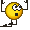# Calculating flow rate through turbine

• Slendermann

#### Slendermann

Hi guys,

First post on this forum. Greetings from the Netherlands :)

I am currently in the process of designing a turbine used in locks in several canals. The turbine is powered when the lock chamber is being emptyed during the lockage.

The goal is to calculate the water level in the lock chamber and the flow rate through the turbine at any given time assuming the turbine has a fixed power level and all of the water flows through it. I've made an attempt at finding a solution, but I would like to know if I'm on the right track.

The water level in the lock chamber decreases as water is flowing out of it. The flow rate and thus the power produced by the turbine decreases as time progresses. However, as the turbine is converting energy into electricity, it in turn is decreasing the flow rate even further. Correct?

From Bernoulli we know:

g*hl = v2/2+g*ht

hl is the water level in the lock chamber or "elevation head", and hk is the head loss produced by the turbine.

We also know:

P = ρ*Q*g*ht
ht=P/(ρ*A*v*g)

(Head loss produced by the turbine equals power divided by the density of water times the surface area of the pipe times the flow speed times acceleration due to gravity)

This gives:

g*hl = v2/2+P/(ρ*A*v)

Which can be written as:

0.5*ρ*A*v3 - ρ*A*hl*g*v + P = 0

This formula can then be used to iterate hl and the flow rate.

Is this formula describing the flow rate A*v and the water level hl correctly for this scenario? Am I missing something?

Thanks guys!

Slendermann

The power $P$ would be:
$$P = \eta \Delta p_o Q$$
where:
$\Delta p_o$ is the pressure drop across the turbine ($= \rho g h_l - p_{o\ out}$);
$Q$ is the flow rate;
$\eta$ is the turbine efficiency, which will depend on the turbine design and - most probably - on $\Delta p_o$ and $Q$ as well.

The proper use of the Bernoulli equation would be for the exit conditions of the turbine:
$$p_{o\ out} = p_{out} + \frac{1}{2}\rho v_{out}^2$$
$$p_{o\ out} = p_{out} + \frac{1}{2}\rho \left(\frac{Q}{A_{out}}\right)^2$$
So the water level - flow rate relation is:
$$P = \eta \left(\rho g h_l - p_{out} - \frac{1}{2}\rho \left(\frac{Q}{A_{out}}\right)^2\right)Q$$
Or:
$$\frac{\rho}{2A_{out}^2}Q^3 - \left(\rho g h_l - p_{out} \right)Q + \frac{P}{\eta} = 0$$
I doubt that you will be able to keep a constant power, though.

Last edited:
Thank you for the reply. If let's say the water flow is constant (controlled via a valve), the only variable left to determine the power output is the outgoing pressure. Is this something you can regulate without changing the water flow? If not, and the outgoing pressure is still linked to the power output, I still cannot find the answer to my question.

In other words: Can pout be substituted by known variables in these equations?

Just use a turbine design which is least sensitive to supply head variation and then take what comes from the coupled generator .

Use electronics and short term battery storage to give a regulated final output .

$p_{out}$ will depend on your exit conditions. If you are discharging into atmosphere, then it will be the atmospheric pressure. If you're discharging at the bottom of a second container, it would be $\rho g h_{2}$.

Ok! Thanks guys, you have been a great help!You will find some useful information on sites dealing with tidal barrage power generation .